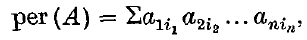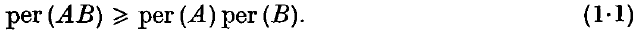Home

Permanent of the product of doubly stochastic matrices

Extract

If A = [aij] is an n × n matrix, the permanent of A is the scalar valued function of A defined bywhere the summation extends over all permutations (i1, i2, …, in) of the integers 1, 2, …, n. If we assume that A is a non-negative matrix (that is, that A has non-negative entries) then we come upon an extremely interesting situation. Much work has been done in finding significant upper bounds for the permanent and permanental minors of A (see, e.g. (2, 4–7) in (5) an excellent bibliography is given). If B = [bij] is another n × n non-negative matrix, then we may form the product AB and consider the permanent of this matrix. Unlike the determinant, the permanent is not a multiplicative function. In our circumstances here, however, it is easy to verify and indeed a proof was written down in (1) thatHide All

Metrics

Full text viewsFull text views reflects the number of PDF downloads, PDFs sent to Google Drive, Dropbox and Kindle and HTML full text views.

Total number of HTML views: 0
Total number of PDF views: 0 *Loading metrics...

Abstract viewsAbstract views reflect the number of visits to the article landing page.

Total abstract views: 0 *Loading metrics...

* Views captured on Cambridge Core between <date>. This data will be updated every 24 hours.

Usage data cannot currently be displayed